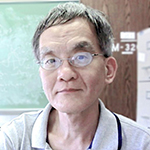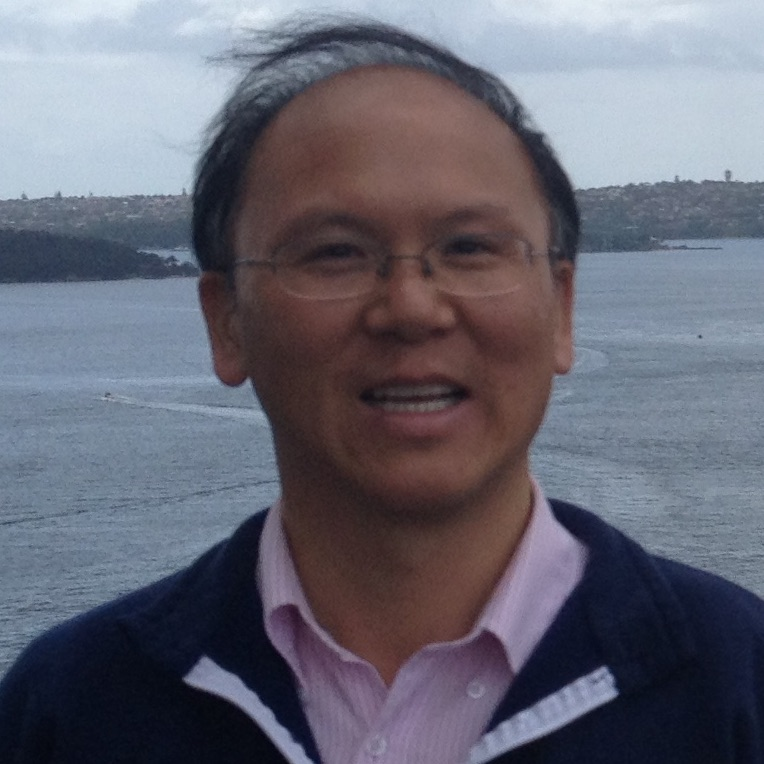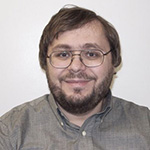# Applied Mathematics

## Research Activity

• There is activity in the area of differential equations, particularly those arising in applications in science and engineering. Research is conducted in both the qualitative theory and the numerical solution of these equations on modern high speed computers. Particular emphasis is on equations arising from continuum mechanics.
• In the area of applied functional analysis, work is done on the application of analysis to problems in tomography and remote sensing.
• In the area of applied and numerical linear algebra the main emphasis is on the solution of large scale problems, computations in stochastic processes, and nonnegative matrices. In particular, there is interest in the development and analysis of new algorithms and their implementation on parallel and vector processing machines.
• Implementation of the Mountain Pass Theorem.
• PDE problems from material science.
• Other areas that are represented include mathematical physics and space-time structure.

## Members#### Yung Choi#### Jeffrey Connors#### Maksym Derevyagin#### Dmitriy Leykekhman#### Guozhen Lu